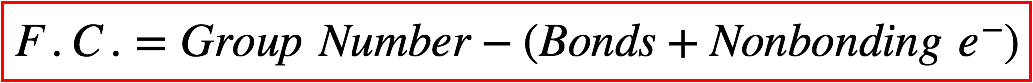# Problem: Draw the best Lewis structure for ClO 4 – and determine the formal charge on chlorine.A) -1B) +1C) 0D) +2E) +3

###### FREE Expert Solution
87% (137 ratings)
###### FREE Expert Solution

We’re being asked to determine the formal charge of chlorine in ClO4

To do so, we need to do the following steps:

Step 1: Determine the central atom in this molecule.

Step 2: Calculate the total number of valence electrons present.

Step 3: Draw the Lewis structure for the molecule.

Step 4: Calculate the formal charge for the indicated atom.

Recall that the formula for the formal charge is:87% (137 ratings)###### Problem Details

Draw the best Lewis structure for ClO  and determine the formal charge on chlorine.

A) -1

B) +1

C) 0

D) +2

E) +3

What scientific concept do you need to know in order to solve this problem?

Our tutors have indicated that to solve this problem you will need to apply the Formal Charge concept. You can view video lessons to learn Formal Charge. Or if you need more Formal Charge practice, you can also practice Formal Charge practice problems.

What is the difficulty of this problem?

Our tutors rated the difficulty ofDraw the best Lewis structure for ClO 4 – and determine the ...as low difficulty.

How long does this problem take to solve?

Our expert Chemistry tutor, Jules took 2 minutes and 50 seconds to solve this problem. You can follow their steps in the video explanation above.

What professor is this problem relevant for?

Based on our data, we think this problem is relevant for Professor Tang's class at USF.